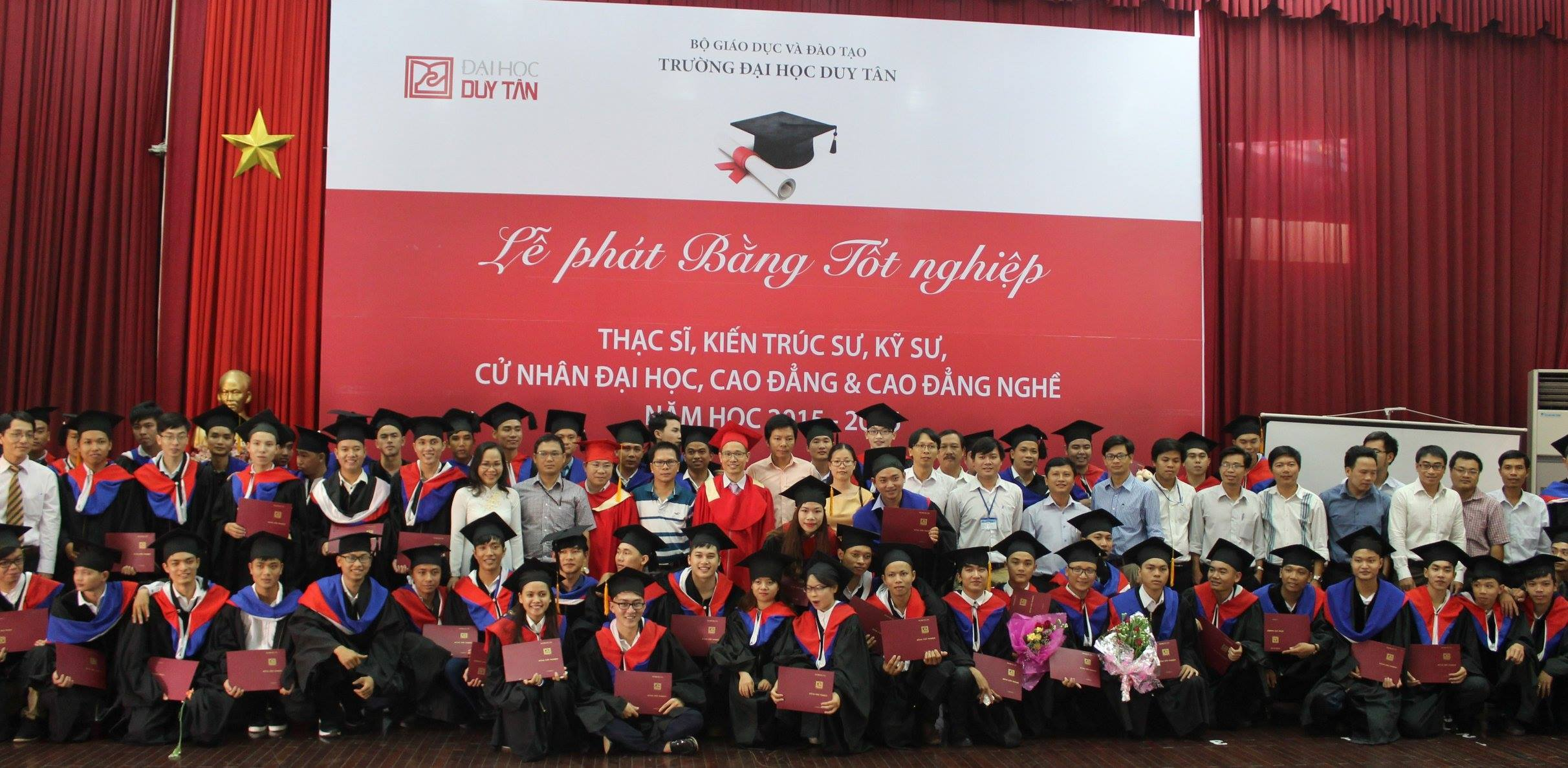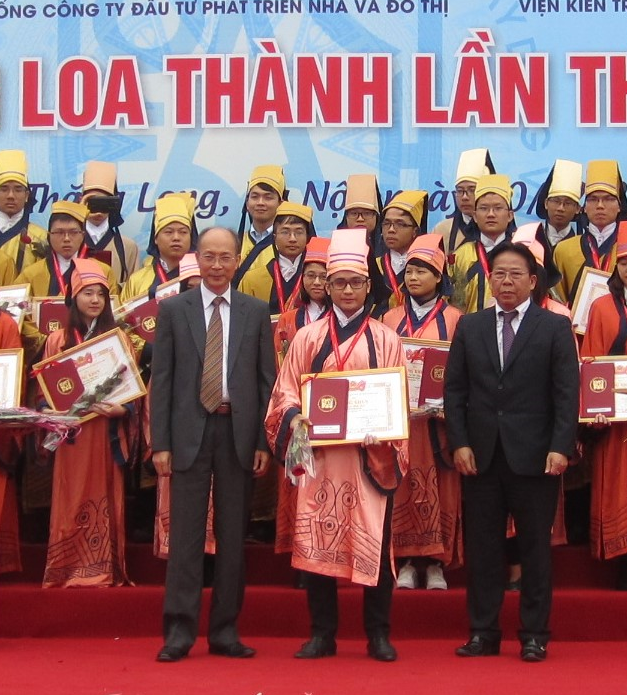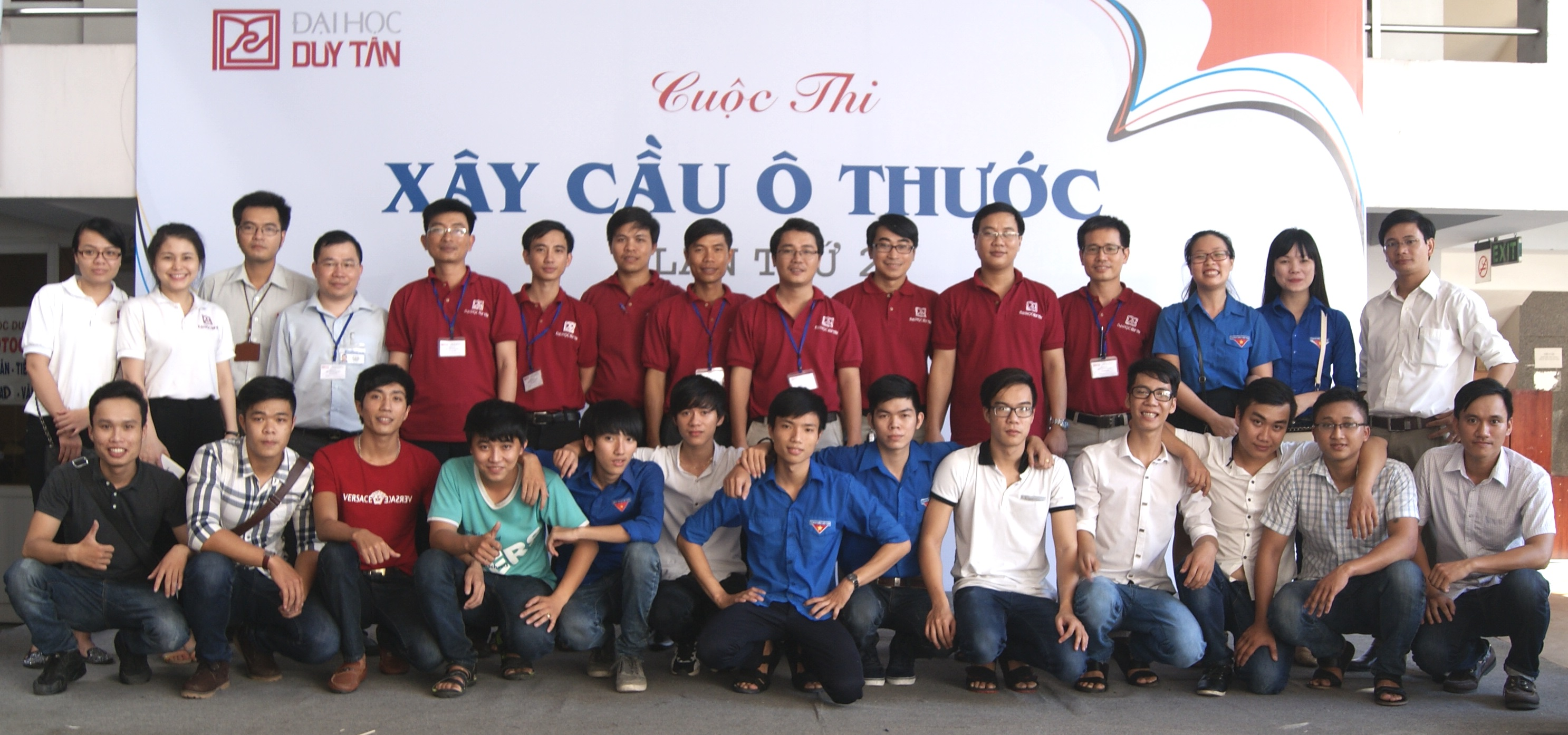Tài liệu học tập

### Bài tập SBVL (Tiếng Anh) 01

• Đăng bởi: lethithanhbinh
• Sinh viên
• 18/04/2019

1. The 4-mm-diameter cable BC is made of a steel with E = 200GPa. Knowing that the maximum stress in the cable must not exceed 190 MPa and that the elongation of the cable must not exceed 6 mm, find the maximum load P that can be applied as shown Fig 1.

1. Both portions of the rod ABC are made of an aluminum for which E = 70 GPa (Fig 2). Knowing that the magnitude of P is 4 kN, determine (a) the value of Q so that the deflection at A is zero, (b) the corresponding deflection of B.

1. The rod ABC is made of an aluminum for which E = 70 GPa (Fig 3). Knowing that P = 6 kN and Q = 42 kN, determine the deflection of (a) point A, (b) point B.

1. A torque T = 3 kN.m is applied to the solid bronze cylinder shown Fig 4. Determine (a) the maximum shearing stress, (b) the shearing stress at point D, which lies on a 15-mm-radius circle drawn on the end of the cylinder, (c)the percent of the rque carried By the portion of the cylinder within the 15-mm radius.

1. Knowing that each of the shafts AB, BC, and CD consists of a solid circular rod Fig 5, determine (a) the shaft in which the maximum shearing stress occurs, (b)the magnitude of that stress.

Tải file đính kèm:Phát bằng tốt nghiệpPhan Minh TiếnHoạt động ngoại khóa